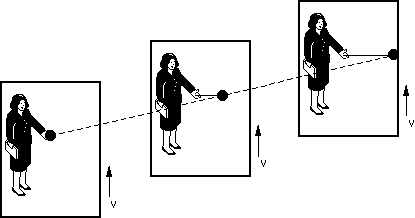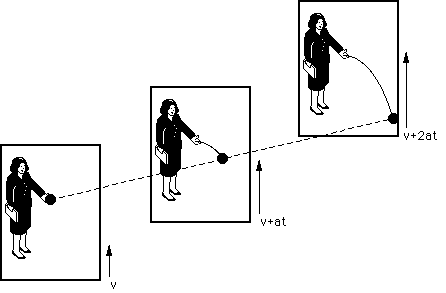The Equivalence PrincipleImagine you are riding in a rocket through space at constant velocity. You throw a ball horizontally. There is no gravity, so the ball moves straight across to the opposite wall (solid line). Someone watching you fly past will see the ball moving in a straight line, up and to the right (dashed line).

Special Relativity applies because the frames are inertial.Now, your rocket is accelerating at a constant rate, a. In the inertial frame, the ball is still moves on a straight line (dashed line). But, in the accelerating frame, the motion is on a curved trajectory (solid line). The rocket is moving faster as time goes on, so it "runs away" from the ball. The trajectory is a parabola, the same path the ball would follow in a constant gravitational field (like the Earth's). Everything in the accelerating rocket experiences a downward force, including the occupant. It's just like standing on Earth, if a=g (the acceleration of gravity on Earth).

Einstein generalized this to the Equivalence Principle, which says (roughly) that a gravitational field is equivalent to an accelerating reference frame. It is sometimes expressed as the equivalence of gravitational and inertial mass, namely that objects with the same inertia (resistance to acceleration) experience the same gravitational force. This is the foundation of General Relativity.

See QuickTime animations of the motion of the ball in accelerated and inertial frames.

Back to the Syllabus.Home